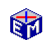The Demand for Labor

To maximize profits, firms choose an optimal demand for factors of production and an optimal quantity of output.  This EconModel application focuses on the profit maximizing demand for a factor of production, which in this case is labor.Model LinkThe Demand for Labor
Printable PDF Exercises

This analysis is closely related to the Average Cost / Marginal Cost analysis of the optimal quantity of output.  Indeed, given that capital is taken as fixed, choosing the optimal demand for labor and choosing the optimal quantity of output both lead to the same optimal level of production.

The basic analysis for this application leads you through a sequence of steps from the point of view of demand for a factor of production:

• Draw Graphs of Production and Profits
• Maximize Profits Given a Wage Rate
• Derive the Labor Demand Curve
• Analyze Effects of Price Changes
• Analyze Effects of Technology Changes

A second sequence of steps shows the correspondence between the profit-maximizing labor demand curve and the average cost/marginal cost view of the profit-maximizing quantity of output.

Classic Economic Models

Microeconomics

Introduction
Overview of Micro Models

Macroeconomics

Introduction
Overview of Macro Models

Resources